# Texas Go Math Grade 1 Lesson 4.6 Answer Key Put Together Numbers to 10

Refer to our Texas Go Math Grade 1 Answer Key Pdf to score good marks in the exams. Test yourself by practicing the problems from Texas Go Math Grade 1 Lesson 4.6 Answer Key Put Together Numbers to 10.

## Texas Go Math Grade 1 Lesson 4.6 Answer Key Put Together Numbers to 10

Essential Question
How can you show ways to make a number?
Explanation:
We have to write partners for the number
4 partners are 1 and 3, 2 and 2, 3 and 1

Explore
Use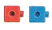to show all the ways to make 5. Color to show your work.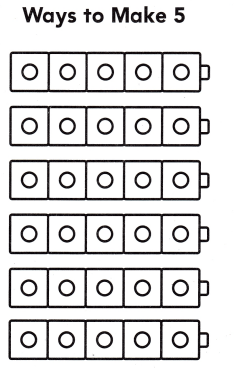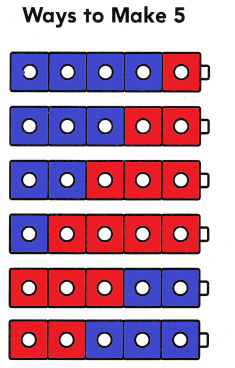Explanation:
The above picture shows how grand ma shares
the ways to make 5 flowers

For The Teacher

• Read the following problem, and have children show all the ways to solve the problem. Grandma has 5 flowers. How many can she put in her red vase, and how many in her blue vase?

Math Talk
Mathematical Processes
How do you know you showed all the ways? Explain.
Explanation:
The process of numbers will be repeated
after once it is completed.

Model and Draw

Now Grandma has 9 flowers. How many can she put in her red vase, and how many in her blue vase?

Question 1.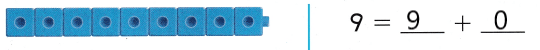Explanation:
9 + 0 = 9
grand ma doesn’t shared any flowers in red vase

Question 2.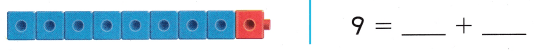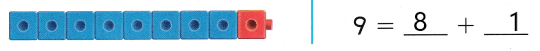Explanation:
8 + 1  = 9
grand ma shared one flowers in red vase

Share and Show

UseColor to show ways to make 9. Complete the addition sentences.

Question 3.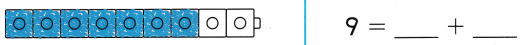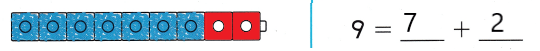Explanation:
The partners of 9 are 2 and 7
blue = 7
red = 2

Question 4.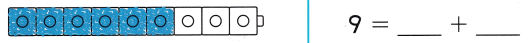Explanation:
The partners of 9 are 6 and 3
blue = 6
red = 3

Question 5.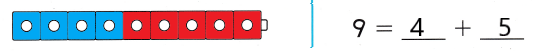Explanation:
The partners of 9 are 4 and 5
blue = 4
red = 5

Question 6.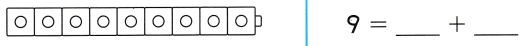Explanation:
The partners of 9 are 3 and 6
blue = 3
red = 6

Question 7.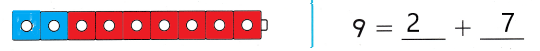Explanation:
The partners of 9 are 2 and 7
blue = 2
red = 7

Question 8.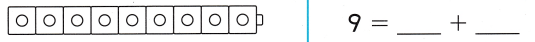Explanation:
The partners of 9 are 1 and 8
blue = 1
red = 8

Question 9.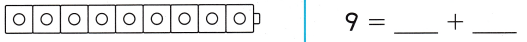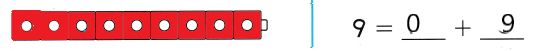Explanation:
The partners of 9 are 0 and 9
blue = 0
red = 9

Question 10.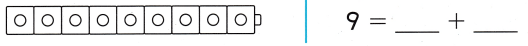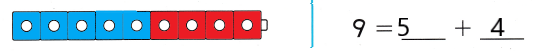Explanation:
The partners of 9 are 5 and 4
blue = 5
red = 4

Problem Solving

Use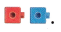Color to show ways to make 10. Complete the addition sentences.

Question 11.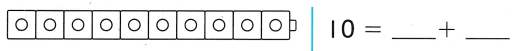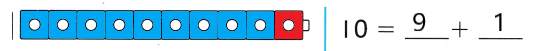Explanation:
The partners of 10 are 9 and 1
blue = 9
red = 1

Question 12.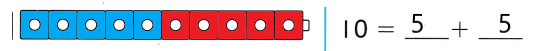Explanation:
The partners of 10 are 5 and 5
blue = 5
red = 5

Question 13.Explanation:
The partners of 10 are 6 and 4
blue = 6
red = 4

Question 14.Explanation:
The partners of 10 are 3 and 7
blue = 3
red = 7

Question 15.
H.O.T. Multi-Step Use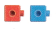to show all the other ways to make 10. Use your MathBoard to draw the other ways. Then write the addition sentence.

Look back at Exercises 11-14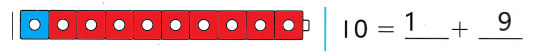Explanation:
The partners of 10 are 1 and 9
blue = 1
red = 9Explanation:
The partners of 10 are 4 and 6
blue = 4
red = 6

Question 16.
H.O.T. Zach has 6 rocks. He places some in a box and some in a bag. Draw two ways he can place the rocks.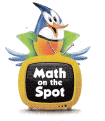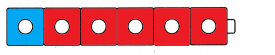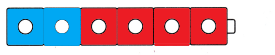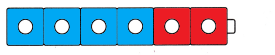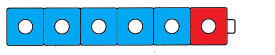Explanation:
1 + 5 = 6
2 + 4 = 6
3 + 3 = 6
4 + 2 = 6
5 + 1 = 6

UseChoose the correct answer.

Question 17.
Brown and green frogs are hopping into the pond. There are 9 frogs in all. Which shows a way to make 9?(A) 4 + 5
(B) 3 + 4
(C) 9 + 1
Explanation:
4 + 5 = 9
shows the way to make 9

Question 18.
Display Tabitha writes to show ways to make 8. Which shows a way to make 8?
(A) 4 + 4
(B) 7 + 4
(C) 2 + 4
Explanation:
4 + 4 = 8
Is the way to make 8

Question 19.
Brian writes to show a way to make 10. Which shows a way to make 10?
(A) 7 + 1
(B) 5 + 5
(C) 6 + 1
Explanation:
5 + 5 = 10 shows a way to make 10

Question 20.
Texas Test Prep Use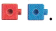Which shows a way to make 7?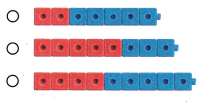Explanation:
2 + 5 = 7
Is the way to make 7

Take Home Activity

• Write 6 = 6 + 0. Model the problem with pennies. Ask your child to make 6 another way. Take turns until you and your child model all of the ways to make 6.Explanation:
1 + 5 = 6
2 + 4 = 6
3 + 3 = 6
4 + 2 = 6
5 + 1 = 6

### Texas Go Math Grade 1 Lesson 4.6 Homework and Practice Answer Key

Use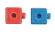Color to show ways to make 10. Complete the addition sentences.

Question 1.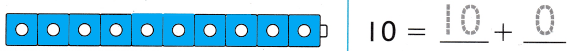Explanation:
The partners of 10 are 10 and 0
blue = 10
red = 0

Question 2.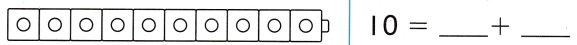Explanation:
The partners of 10 are 9 and 1
blue = 9
red = 1

Question 3.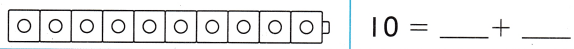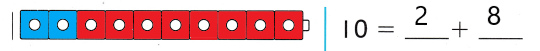Explanation:
The partners of 10 are 2 and 8
blue = 5
red = 5

Question 4.Explanation:
The partners of 10 are 5 and 5
blue = 5
red = 5

Question 5.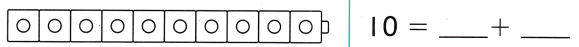Explanation:
The partners of 10 are 6 and 4
blue = 6
red = 4

Question 6.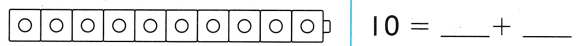Explanation:
The partners of 10 are 0 and 10
blue = 0
red = 10

Question 7.Explanation:
The partners of 10 are 3 and 7
blue = 3
red = 7

Question 8.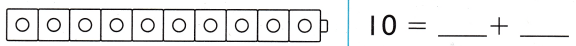Explanation:
The partners of 10 are 8 and 2
blue = 8
red = 2

Question 9.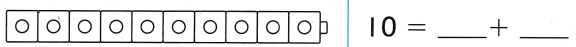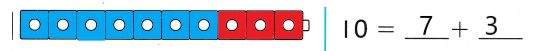Explanation:
The partners of 10 are 7 and 3
blue = 7
red = 3

Question 10.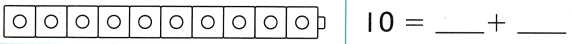Explanation:
The partners of 10 are 4 and 6
blue = 4
red = 6

Question 11.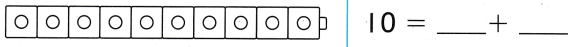Explanation:
The partners of 10 are 1 and 9
blue = 1
red = 9

Texas Test Prep

Lesson Check

Question 5.
The zoo has bears and elephants. There are 7 in all. Which shows a way to make 7?(A) 7 + 1
(B) 2 + 3
(C) 3 + 4
Explanation:
4 + 3 = 7
a way to make 7

Question 6.
Julian writes to show ways to make 6. Which might be a way he writes?
(A) 1 + 3
(B) 3 + 3
(C) 4 + 3
Explanation:
3 + 3 = 6
will  be a way he writes

Question 7.
Which shows a way to make 9?
(A) 5 + 5
(B) 5 + 4
(C) 5 + 2
Explanation:
4 + 5 = 9
A way to make 9

Question 8.
Multi-Step Jaden writes to show ways to make 10. Which might be a way he writes?
(A) 9 + 1
(B) 6 + 2
(C) 1 + 8